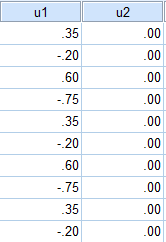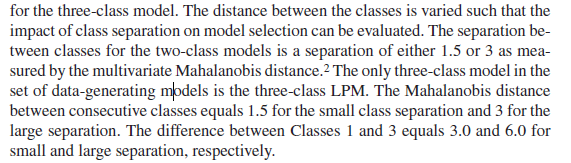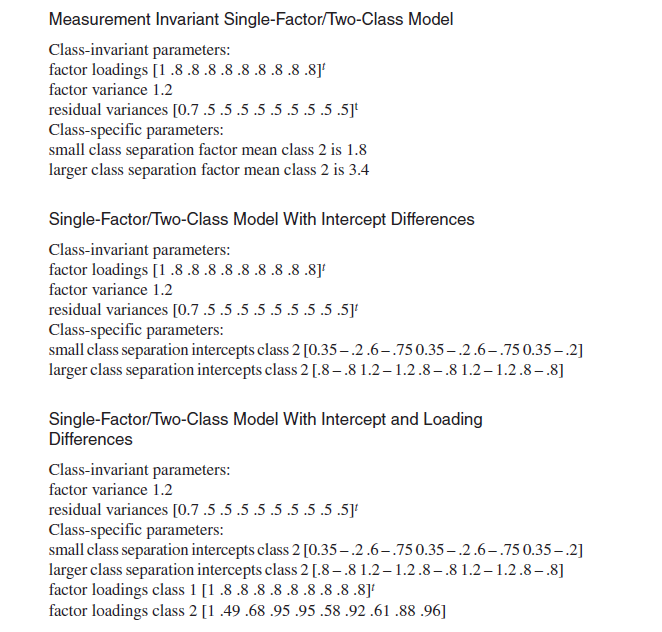## how to calculate the mahalanobis distance with my data(two groups of continuous variable intercept)

I want to calculate the MD which represents the class separation between these two classes.(variable intercepts are shown in the picture attached) .

The intercepts for the first group are 0.35,-0.2,0.6,-0.75,0.35,-0.2,0.6,-0.75,0.35,-0.2

The intercepts for the second group are 0,0,0,0,0,0,0,0,0,0

How to calculate the MD beween these two latent classes(in mixture modeling)? I don't know how to edit the appropriate syntax. Thanks in advance!1 ACCEPTED SOLUTION

Accepted Solutions

## Re: how to calculate the mahalanobis distance with my data(two groups of continuous variable interce

The Euclidean distance formula you are using is the distance between two 10 -dimensional points. The general ED  formula for the distance between points p and q is

ED = sqrt( (p1-q1)**2 + (p2-q2)**2 + ... + (p10 -q10)**2 )

In this case p is the set of 10 X values and q is the center (0,0,0,...0).

So you need to produce a nonsingular 10x10 covariance matrix if you want to compute the Mahalanobis distance.

15 REPLIES 15

## Re: how to calculate the mahalanobis distance with my data(two groups of continuous variable interce

My original syntax as follows:

proc iml;
/* simplest approach: x and center are row vectors */
start mahal1(x, center, cov);
y = (x - center)`; /* col vector */
d2 = y` * inv(cov) * y; /* explicit inverse. Not optimal */
return (sqrt(d2));
finish;

x = {0.35 0，
-0.2 0，
0.6 0，
-0.75 0，
0.35 0，
-0.2 0，
0.6 0，
-0.75 0，
0.35 0，
-0.2 0};  /* test it */
center = {0.015 0};
cov = {0.1225 -0.07 0.21 -0.2625 0.1225 -0.07 0.21 -0.2625 0.1225 -0.07,
-0.07 0.04 -0.12 0.15 -0.07 0.04 -0.12 0.15 -0.07 0.04,
0.21 -0.12 0.36 -0.45 0.21 -0.12 0.36 -0.45 0.21 -0.12,
-0.2625 0.15 -0.45 0.5625 -0.2625 0.15 -0.45 0.5625 -0.2625 0.15,
0.1225 -0.07 0.21 -0.2625 0.1225 -0.07 0.21 -0.2625 0.1225 -0.07,
-0.07 0.04 -0.12 0.15 -0.07 0.04 -0.12 0.15 -0.07 0.04,
0.21 -0.12 0.36 -0.45 0.21 -0.12 0.36 -0.45 0.21 -0.12,
-0.2625 0.15 -0.45 0.5625 -0.2625 0.15 -0.45 0.5625 -0.2625 0.15,
0.1225 -0.07 0.21 -0.2625 0.1225 -0.07 0.21 -0.2625 0.1225 -0.07,
-0.07 0.04 -0.12 0.15 -0.07 0.04 -0.12 0.15 -0.07 0.04};
md1 = mahal1(x, center, cov);
print md1;

## Re: how to calculate the mahalanobis distance with my data(two groups of continuous variable interce

Here is the output, the program presented error messages:

1 OPTIONS NONOTES NOSTIMER NOSOURCE NOSYNTAXCHECK;
42 ;
43 proc iml;
44 /* simplest approach: x and center are row vectors */
45 start mahal1(x, center, cov);
46 y = (x - center)`;
46 ! /* col vector */
47 d2 = y` * inv(cov) * y;
47 ! /* explicit inverse. Not optimal */
48 return (sqrt(d2));
49 finish;
NOTE: Module MAHAL1 defined.
50
51 x = {0.35 0，
ERROR: Mixing character with numeric in matrix literal at line=51 col=12.
52 -0.2 0，
53 0.6 0，
54 -0.75 0，
55 0.35 0，
56 -0.2 0，
57 0.6 0，
58 -0.75 0，
59 0.35 0，
60 -0.2 0};
60 ! /* test it */
61 center = {0.015 0};
62 cov = {0.1225 -0.07 0.21 -0.2625 0.1225 -0.07 0.21 -0.2625 0.1225 -0.07,
63 -0.07 0.04 -0.12 0.15 -0.07 0.04 -0.12 0.15 -0.07 0.04,
64 0.21 -0.12 0.36 -0.45 0.21 -0.12 0.36 -0.45 0.21 -0.12,
65 -0.2625 0.15 -0.45 0.5625 -0.2625 0.15 -0.45 0.5625 -0.2625 0.15,
66 0.1225 -0.07 0.21 -0.2625 0.1225 -0.07 0.21 -0.2625 0.1225 -0.07,
67 -0.07 0.04 -0.12 0.15 -0.07 0.04 -0.12 0.15 -0.07 0.04,
68 0.21 -0.12 0.36 -0.45 0.21 -0.12 0.36 -0.45 0.21 -0.12,
69 -0.2625 0.15 -0.45 0.5625 -0.2625 0.15 -0.45 0.5625 -0.2625 0.15,
70 0.1225 -0.07 0.21 -0.2625 0.1225 -0.07 0.21 -0.2625 0.1225 -0.07,
71 -0.07 0.04 -0.12 0.15 -0.07 0.04 -0.12 0.15 -0.07 0.04};
72 md1 = mahal1(x, center, cov);
ERROR: (execution) Matrix has not been set to a value.

operation : - at line 46 column 11
operands : x, center

x 0 row 0 col (type ?, size 0)

center 1 row 2 cols (numeric)

0.015 0

statement : ASSIGN at line 46 column 4
traceback : module MAHAL1 at line 46 column 4

NOTE: Paused in module MAHAL1.
73 print md1;
ERROR: Matrix md1 has not been set to a value.

statement : PRINT at line 73 column 1
74 ;
75 OPTIONS NONOTES NOSTIMER NOSOURCE NOSYNTAXCHECK;
85 ;

## Re: how to calculate the mahalanobis distance with my data(two groups of continuous variable interce

I would recommend that you move your question to the IML forum here:

https://communities.sas.com/t5/SAS-IML-Software-and-Matrix/bd-p/sas_iml

## Re: how to calculate the mahalanobis distance with my data(two groups of continuous variable interce

As of SAS/IML 12.1, which shipped in August 2012 as part of SAS 9.3m2, the MAHALANOBIS function is distributed as part of SAS/IML software. You do not need to define your own function.

The doc has an example. For your example, you list a 10x10 covariance matrix.  That means that the CENTER vector should be a 1x10 row matrix and the X matrix should have 10 columns, not 10 rows.  Also, the covariance matrix must be nonsingular. Yours appears to be singluar.

## Re: how to calculate the mahalanobis distance with my data(two groups of continuous variable interce

Thanks, Dr. Wicklin! I have read the article concerning the calculation of MD within SAS before, but I am so sorry that I don't truly understand what does the "center" mean in SAS.

In your blog, the article says"Given an observation x from a multivariate normal distribution with mean μ and covariance matrix Σ, the squared Mahalanobis distance from x to μ is given by the formula d2 = (x - μ)T Σ -1 (x - μ). You can use this definition to define a function that returns the Mahalanobis distance for a row vector x, given a center vector (usually μ or an estimate of μ) and a covariance matrix:

In my word, the center vector in my example is the 10 variable intercepts of the second class, namely 0,0,0,0,0,0,0,0,0,0.

I know I am wrong, but don't know  what is wrong. I hope that you can help me with my problem. Thank you!

proc iml;
/* simplest approach: x and center are row vectors */
start mahal1(x, center, cov);
y = (x - center)`; /* col vector */
d2 = y` * inv(cov) * y; /* explicit inverse. Not optimal */
return (sqrt(d2));
finish;

x = {0.35 -0.2 0.6 -0.75 0.35 -0.2 0.6 -0.75 0.35 -0.2,
0 0 0 0 0 0 0 0 0 0}; /* test it */
center = {0 0 0 0 0 0 0 0 0 0 0};
cov = {0.1225 -0.07 0.21 -0.2625 0.1225 -0.07 0.21 -0.2625 0.1225 -0.07,
-0.07 0.04 -0.12 0.15 -0.07 0.04 -0.12 0.15 -0.07 0.04,
0.21 -0.12 0.36 -0.45 0.21 -0.12 0.36 -0.45 0.21 -0.12,
-0.2625 0.15 -0.45 0.5625 -0.2625 0.15 -0.45 0.5625 -0.2625 0.15,
0.1225 -0.07 0.21 -0.2625 0.1225 -0.07 0.21 -0.2625 0.1225 -0.07,
-0.07 0.04 -0.12 0.15 -0.07 0.04 -0.12 0.15 -0.07 0.04,
0.21 -0.12 0.36 -0.45 0.21 -0.12 0.36 -0.45 0.21 -0.12,
-0.2625 0.15 -0.45 0.5625 -0.2625 0.15 -0.45 0.5625 -0.2625 0.15,
0.1225 -0.07 0.21 -0.2625 0.1225 -0.07 0.21 -0.2625 0.1225 -0.07,
-0.07 0.04 -0.12 0.15 -0.07 0.04 -0.12 0.15 -0.07 0.04};
md1 = mahal1(x, center, cov);
print md1;

## Re: how to calculate the mahalanobis distance with my data(two groups of continuous variable interce

The 'center' matrix is an estimate of the center of the population. for example, the center is often taken to the be the sample mean of the data, just as the covariance estimate is the sample covariance.

In the code that you posted, there are two things wrong:

1. The Center vector has 11 elements, not 10.

2. The covariance matrix is singular. The covariance must be nonsingular to compute the Mahalanobis distance.

For demonstration purposes, the following code adds a multiple of the identity matrix to your covariance. In real life, you'll have to  figure out how to get a nonsingular matrix.  Notice that I call the built-in MAHALANOBIS function:

``````proc iml;

x = {0.35 -0.2 0.6 -0.75 0.35 -0.2 0.6 -0.75 0.35 -0.2};
center = {0 0 0 0 0 0 0 0 0 0};
cov = {0.1225 -0.07 0.21 -0.2625 0.1225 -0.07 0.21 -0.2625 0.1225 -0.07,
-0.07 0.04 -0.12 0.15 -0.07 0.04 -0.12 0.15 -0.07 0.04,
0.21 -0.12 0.36 -0.45 0.21 -0.12 0.36 -0.45 0.21 -0.12,
-0.2625 0.15 -0.45 0.5625 -0.2625 0.15 -0.45 0.5625 -0.2625 0.15,
0.1225 -0.07 0.21 -0.2625 0.1225 -0.07 0.21 -0.2625 0.1225 -0.07,
-0.07 0.04 -0.12 0.15 -0.07 0.04 -0.12 0.15 -0.07 0.04,
0.21 -0.12 0.36 -0.45 0.21 -0.12 0.36 -0.45 0.21 -0.12,
-0.2625 0.15 -0.45 0.5625 -0.2625 0.15 -0.45 0.5625 -0.2625 0.15,
0.1225 -0.07 0.21 -0.2625 0.1225 -0.07 0.21 -0.2625 0.1225 -0.07,
-0.07 0.04 -0.12 0.15 -0.07 0.04 -0.12 0.15 -0.07 0.04};

cov = cov + I(10);   /* HACK: make the cov matrix nonsingular for demonstration */
md1 = mahalanobis(x, center, cov);
print md1;

``````

## Re: how to calculate the mahalanobis distance with my data(two groups of continuous variable interce

Hello again! With the default MD function and the previous syntax, the MD value is 0.83. But in the simulation study, the author fixed the MD value at 1.5 for small separation between the 2 classes. (As you can see in the 2 pictures attached) Thank you for your light on this difference!## Re: how to calculate the mahalanobis distance with my data(two groups of continuous variable interce

"For demonstration purposes, the following code adds a multiple of the identity matrix to your covariance. In real life, you'll have to  figure out how to get a nonsingular matrix."

Also the program says

``/* HACK: make the cov matrix nonsingular for demonstration */``

In other words, I am not claiming that the MD is 0.83.  You have provided a singular matrix, which means that the MD is undefined. I modified your covariance matrix in order to show you how to calculate the MD. You won't match someone else's results unless you use the same inputs (mean vector and covariance matrix) that they used.

## Re: how to calculate the mahalanobis distance with my data(two groups of continuous variable interce

Yes, the covariance matrix in fact should be a 2*2 matrix because the sample has 2 dimensions(2 groups of observed variable intercepts).

cov = {0.2589 0,

0 0};

The x should be

x = {0.35 -0.2 0.6 -0.75 0.35 -0.2 0.6 -0.75 0.35 -0.2};

But the center. You said the center is the sample mean of the data.

The center should be {0.015,0};

But this syntax still did not work. I am a green hand in statistics. Thank you for your help!

proc iml;

x = {0.35 -0.2 0.6 -0.75 0.35 -0.2 0.6 -0.75 0.35 -0.2};

center = {0.015 0};

cov = {0.2589 0,

0 0};

md1 = mahalanobis(x, center, cov);

print md1;

## Re: how to calculate the mahalanobis distance with my data(two groups of continuous variable interce

Let p be the number of variables in your problem.

Then

• X should be a matrix that has p columns.
• 'center' should be a 1 x p row vector.
• the covariance matrix should be a symmetric nonsingular p x p matrix.

In your problem, do you have 2 variables or 10?

## Re: how to calculate the mahalanobis distance with my data(two groups of continuous variable interce

The number of variables p is 2 in my example. The intercepts of the two latent classes specify the class separation. But in my example, the covariance beween u1 and u2 is 0. The MD simplifies to Euclidean distance. Therefore I can calculate the ED manually and get the 1.5 value. But in SAS with the built-in MD function, I don't know how to range the sample values in matrix X. Thank you! Here is my syntax. proc iml; x = {0.35 -0.2 0.6 -0.75 0.35 -0.2 0.6 -0.75 0.35 -0.2, 0 0 0 0 0 0 0 0 0 0}; center = {0.015 0}; cov = {0.2589 0, 0 0}; md1 = mahalanobis(x, center, cov); print md1;

## Re: how to calculate the mahalanobis distance with my data(two groups of continuous variable interce

Sorry to repeat myself, but you still do not have the dimensions correct.

1. If the mean is 1x2 and the covariance matrix is 2x2, then the X matrix must have two columns.

2. The covariance matrix must be nonsingular. Your matrix cov = {0.2589 0, 0 0} is singular.

3. If you expect to get one "distance" (you say the answer is 1.5), then X would have 1 row.  If you specify 10 rows, you are saying that there are 10 points and you want to know the distance from each point to the center.

If you show the Euclidean calculation (for which you claim you get the correct  answer) that might help us to make sense of what you are trying to do.

## Re: how to calculate the mahalanobis distance with my data(two groups of continuous variable interce

Here is the Euclidean calculation with respect to my example, Euclidean distance = sqrt[0.35*2+(-0.2)*2+0.6*2+(-0.75)*2+0.35*2+(-0.2)*2+0.6*2+(-0.75)*2+0.35*2+(-0.2)*2] = sqrt(2.3325) = 1.53 I am not very familiar with sas program and may confuse the definitions. Thank you for your support and understanding!

## Re: how to calculate the mahalanobis distance with my data(two groups of continuous variable interce

The Euclidean distance formula you are using is the distance between two 10 -dimensional points. The general ED  formula for the distance between points p and q is

ED = sqrt( (p1-q1)**2 + (p2-q2)**2 + ... + (p10 -q10)**2 )

In this case p is the set of 10 X values and q is the center (0,0,0,...0).

So you need to produce a nonsingular 10x10 covariance matrix if you want to compute the Mahalanobis distance.

From The DO Loop